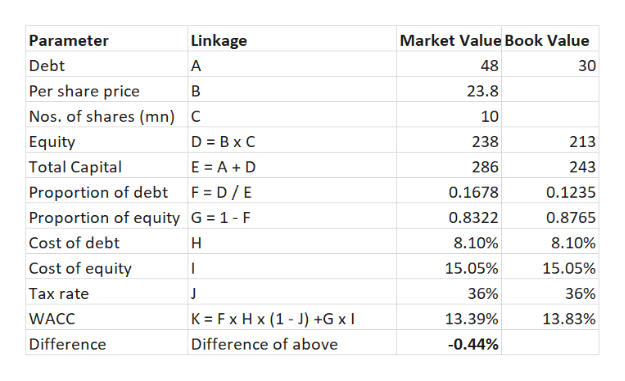ABC Trucking's balance sheet shows a total of noncallable \$30 million long-term debt with a coupon rate of 6.80% and a yield to maturity of 8.10%. This debt currently has a market value of \$48 million. The balance sheet also shows that the company has 10 million shares of common stock, and the book value of the common equity  is \$213.00 million. The current stock price is \$23.80 per share; stockholders' required return, rs, is 15.05%; and the firm's tax rate is 36.00%. The CFO thinks the WACC should be based on market value weights, but the president thinks book weights are more appropriate. What is the difference between the WACCs using market value and the book value?Work with at least 4 decimals and round your final answer to two decimal places. For example, if your answer is \$345.6671 round as 345.67 and if your answer is .05718 or 5.7182% round as 5.72. A. –0.49%B. –0.44%C. –0.46%D. –0.34%E. –0.36%

Question
ABC Trucking's balance sheet shows a total of noncallable \$30 million long-term debt with a coupon rate of 6.80% and a yield to maturity of 8.10%. This debt currently has a market value of \$48 million. The balance sheet also shows that the company has 10 million shares of common stock, and the book value of the common equity  is \$213.00 million. The current stock price is \$23.80 per share; stockholders' required return, rs, is 15.05%; and the firm's tax rate is 36.00%. The CFO thinks the WACC should be based on market value weights, but the president thinks book weights are more appropriate. What is the difference between the WACCs using market value and the book value?

Work with at least 4 decimals and round your final answer to two decimal places. For example, if your answer is \$345.6671 round as 345.67 and if your answer is .05718 or 5.7182% round as 5.72.

A. –0.49%
B. –0.44%
C. –0.46%
D. –0.34%
E. –0.36%

Hence, the correct answer is th...help_outlineImage TranscriptioncloseLinkage Market Value Book Value Parameter Debt 48 A 30 Per share price В 23.8 Nos. of shares (mn) 10 C D Bx C Equity 238 213 Total Capital E A D 286 243 Proportion of debt F D/E Proportion of equity G 1- F 0.1678 0.1235 0.8322 0.8765 Cost of debt н 8.10% 8.10% Cost of equity 15.05% 15.05% | Tax rate J 36% 36% K Fx Hx (1-J) +G x WACC 13.39% 13.83% Difference Difference of above -0.44% fullscreen

Want to see the full answer?

See Solution

Want to see this answer and more?

Our solutions are written by experts, many with advanced degrees, and available 24/7

See Solution
Tagged in